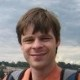# Jitse Niesen

Leeds, United Kingdom

http://www.maths.leeds.ac.uk/~jitse/

I am a lecturer in the School of Mathematics at the University of Leeds. My research specialization is numerical analysis (mostly ordinary differential equations, some partial differential equations, some linear algebra). Most of my programming is related to this; in particular I contribute to the Eigen library (linear algebra, C++). I usually program in Python, C++ or MATLAB. I have been using LaTeX from \pm 1994.

Top Questions